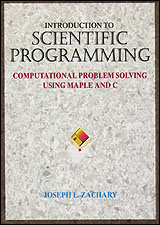### Introduction to Scientific Programming Computational Problem Solving Using: Maple and C Mathematica and C

Author:
Joseph L. Zachary
Online Resources:
Maple/C Version
Mathematica/C Version

# Bisection Method Tutorial

In this tutorial we will explore the bisection method for finding the roots of equations, as explained in Chapter 9.

## Simulation

We will be using a bisection method simulator throughout this tutorial. You can start it by clicking on the following button.

If you see this, then Java is not running in your browser!
An applet would normally go here...

## Finding Roots

This tutorial explores a simple numerical method for finding the root of an equation: the bisection method. The bisection method is discussed in Chapter 9 as a way to solve equations in one unknown that cannot be solved symbolically.

For example, suppose that we would like to solve the simple equation

``` 2
x  = 5
```
To solve this equation using the bisection method, we first manipulate it algebraically so that one side is zero.
``` 2
x  - 5 = 0
```
Finding a solution to this equation is then equivalent to finding a root of the function
```        2
f(x) = x  - 5
```
This function is plotted in the simulation window.

We next find two numbers, a positive guess and a negative guess, so that f(positive guess) is positive and f(negative guess) is negative. In the simulation window, the positive guess is -5 and the negative guess is 1. The point

```(positive guess, f(positive guess))
```
is displayed with a pink dot, and the point
```(negative guess, f(negative guess))
```
is displayed with a red dot. The coordinates of the two dots are displayed at the bottom of the simulation window.

So long as the function whose root we are finding is continuous, there must be at least one root between the positive and negative guesses. The bisection method locates such a root by repeatedly narrowing the distance between the two guesses.

To see the bisection method in action, click on the button labeled "Step". A blue dot will appear on the curve. The x-coordinate of this point is the average of the positive and negative guesses. Because the value of the function at this point is negative, the x-coordinate of the blue dot becomes the new negative guess. The red dot slides over to take the place of the blue dot. Notice that the point where the curve crosses the x-axis remains between the pink and red dots.

Now click on "Step" again. Once again a blue dot appears, but this time it lies on a positive portion of the curve. This time, then, the x-coordinate of the blue dot becomes the new positive guess, and the pink dot slides over to take the place of the blue dot. As before, the point where the curve crosses the x-axis remains between the pink and red dots.

If you repeat this over and over, the red and blue dots will get closer and closer together, but they will never meet. (When the dots get too close together in the simulation to distinguish, you can zoom in by using the mouse to drag a rectangle around the region that you'd like to enlarge. There is also a "Zoom" menu in the menu bar.) At any point of the simulation, the average of the positive and negative guesses, which is displayed as the x-coordinate of the bisection point, will be an approximation to a root of f. The more steps that the simulation performs, the better the approximation will be.

## Exercises

1. You can place the positive and negative guesses by clicking the mouse where you would like the guess to go. Notice that the function displayed in the simulation window has two roots. Can you place the initial guesses so as to find the other root?

2. If you place the positive guess at -5 and the negative guess at 1, how many steps are required until the approximate root is good to three decimal places? (The root near -2 is -2.236067978 to ten digits; the approximate root is the x-coordinate of the bisection point.)

3. If you place the positive guess at -5 and the negative guess at 1, how many steps are required until the absolute value of f at the approximate root is less than one-thousandth? (The value of f at the approximate root is the y-coordinate of the bisection point.)

4. Use the "Function" menu to display the curve for cos(x). Notice that four different roots are displayed. If you make the positive guess less than -3 and the negative guess greater than 1, how many of the roots can you find?

5. Experiment with some of the other functions to get a feel for how the bisection works and for how many steps it takes for it to come up with a good approximation.